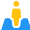# Practical Operational AmplifiersCURRENT – 1. Practical Operational Amplifiers- Introduction

INTRODUCTION

Ideal op-amps were introduced. We presented these from the perspective of a systems engineer who would analyze and design an electronic system. In this chapter, we study a variety of component circuits that make up a typical op-amp. Circuit operation with be demonstrated by circuit simulation using the TINA and TINACloud oflline an online circuit simulators.
We discuss the internal characteristics of the op-amp. First we analyze the difference or differential amplifier, which is the basic building block. Several other internal circuit groups are then discussed.
The chapter then explores the forms of packaging used for op-amps. A discussion of the 741 general purpose op-amp is included for illustrative purposes.
We then modify the ideal op-amp mathematical model by recognizing the changes required to make the model more closely coincide with the real op-amp. Our design method is refined to account for the necessary modifications from the ideal op-amp.
The chapter concludes with a discussion of power audio op-amps.
When you finish studying this chapter, you will:

• Understand the internal operation of the op-amp so you will appreciate its limitations and the circumstances under which it can no longer be considered using the idealized models of Chapter: “Ideal Operational Amplifiers”.
• Be able to calculate a variety of parameters relating to circuits containing operational amplifiers.
• Understand the operation and utility of differential amplifiers.
• Be able to design circuits with balanced inputs and outputs.
• Be aware of the coupling considerations when op-amps operate with multiple inputs.
• Be able to design systems using power audio op-amps.